Get instant live expert help with Excel or Google Sheets“My Excelchat expert helped me in less than 20 minutes, saving me what would have been 5 hours of work!”

#### Post your problem and you’ll get Expert help in seconds.

Your message must be at least 40 characters
Our professional Expert are available now. Your privacy is guaranteed.

# How to Use the Excel IFNA Function

We can use the excel IFNA function to generate a custom result when a formula ought to display the #N/A error. This function doesn’t detect other errors apart from the #N/A error. The steps below will walk through the process.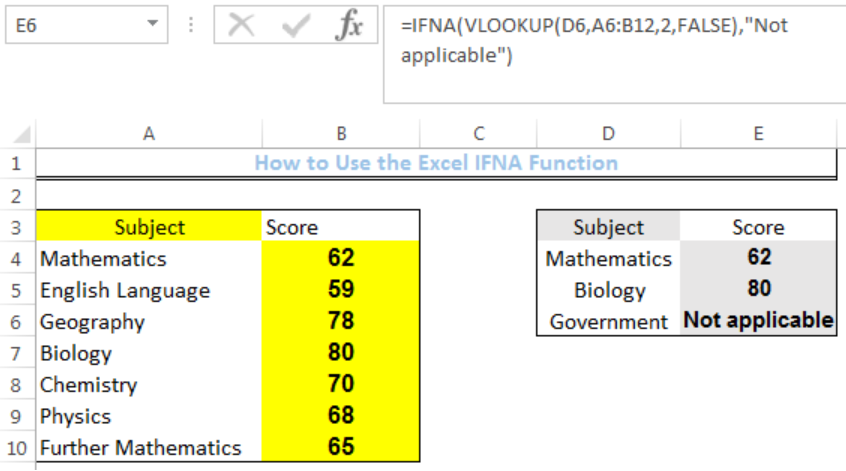Figure 1: How to Use the Excel IFNA Function

## Formula

`=IFNA(VLOOKUP(D4,A4:B10,2,FALSE),"Not applicable")`

## Setting up the Data

• We will set up the data by inputting the SUBJECTS for a student in Column A
• Column B contains the scores for each subject
• Column E is where we want the formula to return the result. We will use a VLOOKUP formula to check for the scores of the subjects if they are in the range (A4:B10)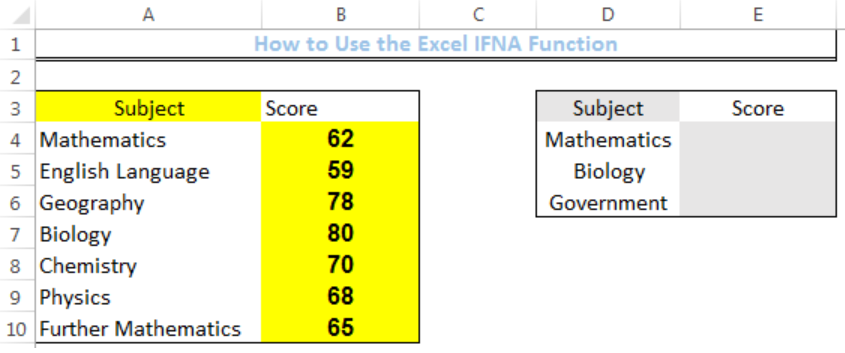Figure 2: Setting up the Data

## Excel IFNA Function

• We will click on Cell E4
• We will insert the formula below into the cell
• `=IFNA(VLOOKUP(D4,A4:B10,2,FALSE),"Not applicable")`
• We will press the enter key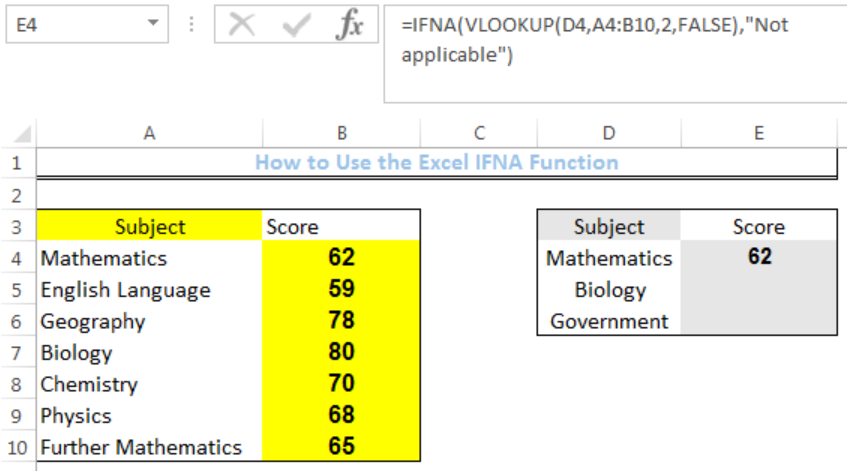Figure 3: Result of VLOOKUP for Cell E4

• We will click on Cell E4 again
• We will double-click on the fill handle (the small plus sign at the bottom right of Cell E4) and drag down to copy the formula into the other cells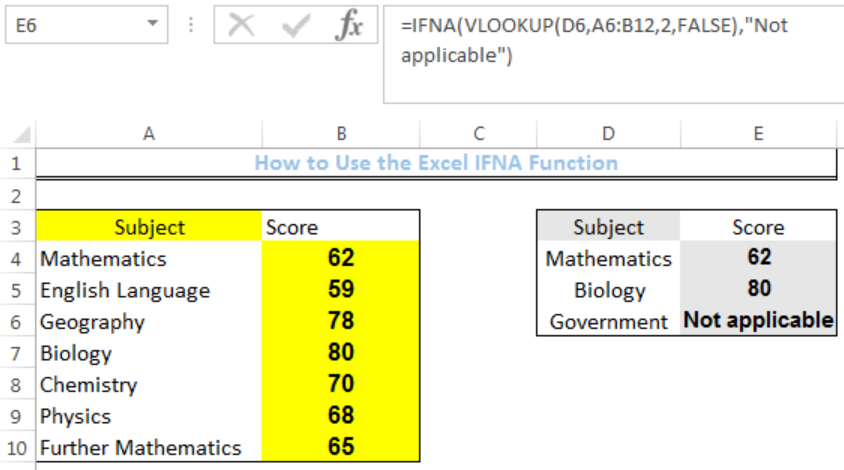Figure 4: Result of Using the IFNA function

## Without the IFNA Function

Without the IFNA Function, the formula for VLOOKUP is: `=VLOOKUP(D4,A4:B10,2,FALSE)`. If we clear the data from Cell E4 to Cell E6 where our result is and input the formula below, our result will be like figure 5.

Formula: `=VLOOKUP(D4,A4:B10,2,FALSE)`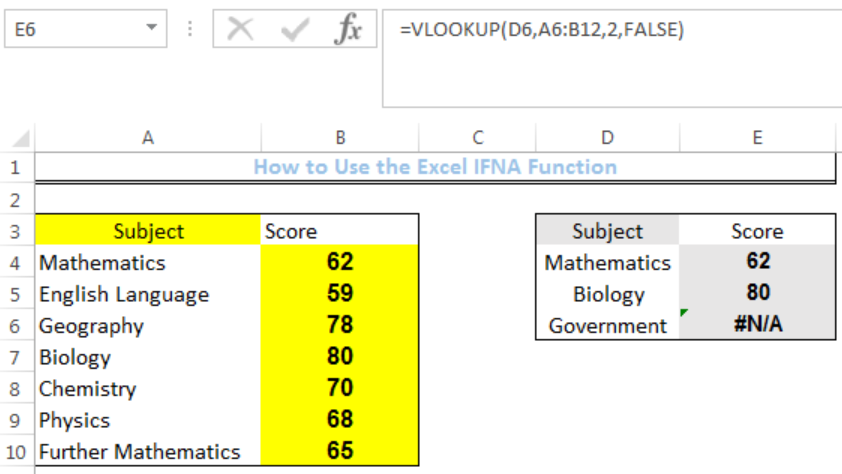Figure 5: Without the IFNA Function

## Instant Connection to an Expert through our Excelchat Service

Most of the time, the problem you will need to solve will be more complex than a simple application of a formula or function. If you want to save hours of research and frustration, try our live Excelchat service! Our Excel Experts are available 24/7 to answer any Excel question you may have. We guarantee a connection within 30 seconds and a customized solution within 20 minutes.

### Did this post not answer your question? Get a solution from connecting with the expert.Another blog reader asked this question today on Excelchat:
Solution examplesHi I'm trying to use fuzzy lookup. Not sure how it's working. I have two strings which are different ('Unemployment Benefits' vs 'Invalid pensioner'), yet getting a similarity index of 0.9474.
Solved by E. E. in 40 minsGood Morning, I am trying to get parts from one column in an excel spread sheet to match up with a parts and cost column from a different excel spread sheet. (Not all parts on each spread sheet are the same)
Solved by S. L. in 49 minsI am trying to perform a vlookup or index match for a large data set. Normally I rely on vlookup but it is giving me data from the wrong column whatever I do. I have tried to move to vlookup and it is doing exactly the same thing. Do you have any tips?
Solved by O. W. in 27 minsI need help with Vlookup- am trying to copy a data from one sheet onto another matching the number but with a column
Solved by C. H. in 20 minsI need to formulate a cell where it will show different values. for example if d2=0 i need e2 to show N. But the range of d2 could be anywhere from 0-60
Solved by I. U. in 29 mins## Subscribe to Excelchat.coAnother blog reader asked this question today on Excelchat: